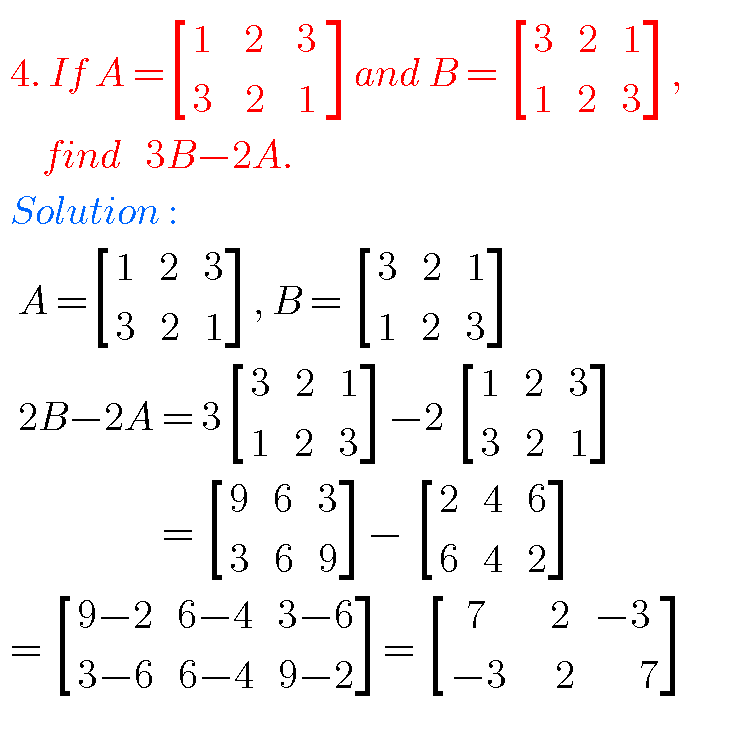## Matrices solutions,Inter Maths 1a chapter 3 solutions

Matrices solutions, Inter maths 1a chapter 3 solutions Mathematics intermediate first year 1a matrices solutions for some problems for examination purpose. Study the text book lesson Matrices very well. Practice the example problems and solutions given in the textbook. Observe the solutions and try them in your own method. You can also see Inter Maths …